Courses

# Dynamic Programming, P & NP Concepts (Basic Level) - 2

## 10 Questions MCQ Test Question Bank for GATE Computer Science Engineering | Dynamic Programming, P & NP Concepts (Basic Level) - 2

Description
This mock test of Dynamic Programming, P & NP Concepts (Basic Level) - 2 for Computer Science Engineering (CSE) helps you for every Computer Science Engineering (CSE) entrance exam. This contains 10 Multiple Choice Questions for Computer Science Engineering (CSE) Dynamic Programming, P & NP Concepts (Basic Level) - 2 (mcq) to study with solutions a complete question bank. The solved questions answers in this Dynamic Programming, P & NP Concepts (Basic Level) - 2 quiz give you a good mix of easy questions and tough questions. Computer Science Engineering (CSE) students definitely take this Dynamic Programming, P & NP Concepts (Basic Level) - 2 exercise for a better result in the exam. You can find other Dynamic Programming, P & NP Concepts (Basic Level) - 2 extra questions, long questions & short questions for Computer Science Engineering (CSE) on EduRev as well by searching above.
QUESTION: 1

### The number of spanning trees for a complete graph with seven vertices is

Solution:

Number of spanning tree possible with n-node = nn - 2.
Given, n = 7
Total number of spanning trees = 75

QUESTION: 2

### If one uses straight two-way merge sort algorithm to sort the following elements in ascending order: 20, 47, 15, 8, 9, 4, 40, 30, 12, 17 Then the order of these elements after second pass of the algorithm is

Solution:

Given array: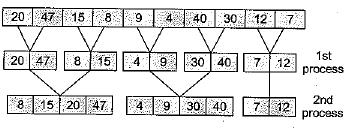So, the order of elements after second pass of the algorithm is 8,15,20,47, 4,9,30,40,12,17,

QUESTION: 3

### Consider the following sorting algorithms: 1. Quicksort 2. Heapsort 3. Mergesort Which of them perform in least time in the worst case?

Solution:

Worst case time complexity of Quick sort = O(n2) when input is already sorted.
Worst case time complexity of Heap sort = O(n log n).
Worst case time complexity of Merge sort = O(n log n).

QUESTION: 4

The number of articulation points of the following graph is: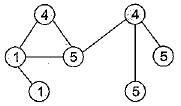Solution:

An articulation point (or cut vertex) is that vertex removing which (along with its edges) disconnects the graph.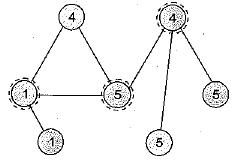Therefore number of articulation points is 3.

QUESTION: 5

Consider the following tree: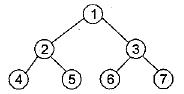If the post order traversal gives ab - cd * + then the label of the nodes 1, 2, 3, ... will be

Solution:

Post order traversal = ab - cd*+. As we know that post order traversal goes in the order of
LEFT CHILD → RIGHT CHILD → PARENT (ROOT)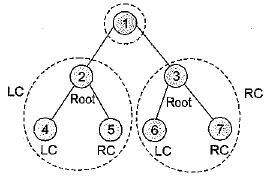Hence, Label of 4, 5, 2 will be a, b, - respectively
Labe! of 6, 7, 3 will be c, d, * respectively
Label of 1 will be +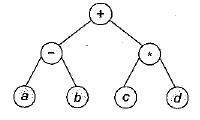QUESTION: 6

The expression tree given in figure evaluates to 1, if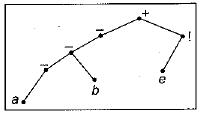1. a = - b and e = 0
2. a = - b and e = 1
3. a = b and e = 0
4. a = b and e = 1

Solution:

The corresponding expression is - (- a - b) + e!.
This is 1 if a = - b and e is either 1 or 0, since 1! = 0! = 1.

QUESTION: 7

Match List-I with List-ll and select the correct answer using the codes given beiow the lists: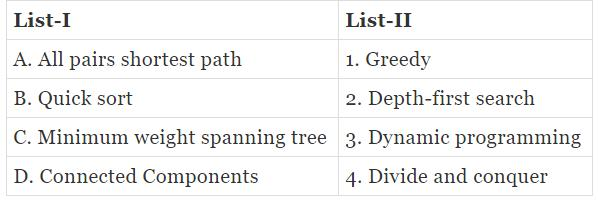Codes: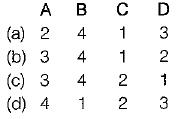Solution:

All pairs shortest path is find using Floyd Warshall algorithm, which is an example of dynamic programming.
• Quick sort and merge sort are example of “divide and conquer” algorithms.
• MST or minimum weight spanning tree is a tree with a subset of edges such that it connects all vertices and summation of edge weight is minimum Prim’s algo and Kruskal’s algorithm are used for this, which are examples of greedy approach.
• By using Depth First Search (DFS) one can easily find set of connected components.

QUESTION: 8

Consider the following tree: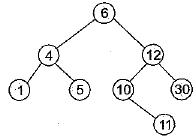If this tree is used for sorting, then a new number 8 should be place as the

Solution:

Since the tree is used for sorting hence taking INORDER TRAVERSAL (Left-Root-Right) we have 8 placed at the left child of the node labeled 10.

QUESTION: 9

The order of a B-tree is defined as the

Solution:

The default order of B-tree is maximum number of pointer in any node.

QUESTION: 10

The depth of a complete binary tree with ‘n’ nodes is (log is to the base two)

Solution:

If the depth is d, the number of nodes n will be 2(d+ 1) - 1.
So, n + 1 = 2(d+1)
or, d = log (n + 1 ) - 1